Thermal Plot PropertyManager

To display this PropertyManager:

1. Run a thermal study.
2. Right-click Results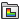and select Define Thermal Plot.

Thermal results are available for nodes only.

Display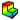Component Select a thermal component to plot. Directions are based on the selected reference geometry.See Thermal Components.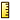Units Select a unit for the thermal plot.
You can control the display of the fringe plot using the Settings PropertyManager.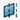Plane, Axis or Coordinate System Select a reference plane or a reference axis to plot directional thermal results. Show as vector plot Available only for resultant temperature gradient (GRADN) and resultant heat flux (HFLUXN). Generates a vector plot where a vector is plotted at each node to show the magnitude and direction of the selected component. You can control the size and density of the vectors in a vector plot using the Vector Plot Options PropertyManager.

Plot Step

Available only for transient thermal studies.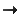Plot for Single Step Select this option to plot the results at the selected solution step.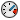Displays the time corresponding to the selected solution step.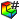Plot Step Sets the solution step number for the plot.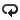Plot Bounds across All Steps Select this option to plot extreme values regardless of the solution step they occur at. Select one of the following:
 Maximum Displays the maximum algebraic values (envelope plot) across all solution steps. Minimum Displays the minimum algebraic values (envelope plot) across all solution steps. Absolute maximum Displays the absolute maximum values (envelope plot) across all solution steps.

Property

 Include title text Type a custom title for the plot. Associate plot with name view orientation Associate a predefined view orientation with the active plot.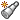Associate with current view Associates the current view with the active plot.